# Count Partitions with Given Difference (DP – 18)

Problem Statement:  Count Partitions with Given Difference

This article will be divided into two parts:

Part 1: Extra edge case for the problem Count Subsets with Sum K

In the problem Count Subsets with Sum K, the problem constraints stated that an array element is greater than 0, so the code we have written there works perfectly for the given constraints.

If the constraints mentioned that an array element can also be equal to 0 and the target sum can also be 0, then that code will fail. To understand it we will take an example:

Let the target arr = [0,0,1] and the target = 1.

The previous code will give us the answer 1 as it first takes the element arr and then finds the answer by picking it. Then from the base condition, we will return 0 ( as the target will become 0 by picking 1). But for this question, the answer will be 4 with the following subsets({0,1},{0,1},{0,0,1} and {1}).

Therefore we need to modify the base conditions in order to handle the changes. These are the base conditions of that problem.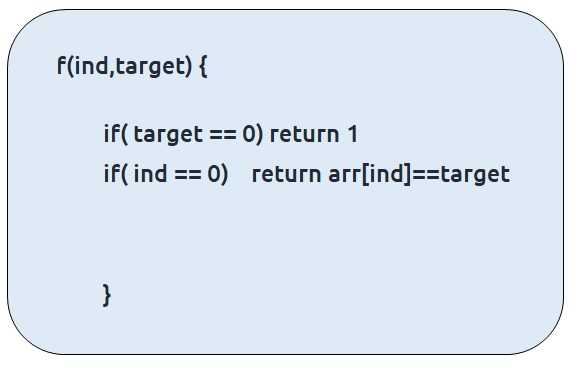• First of all, we will remove target==0 because now when target ==0, there can be many 0s present in the array which needs to be counted in the answer.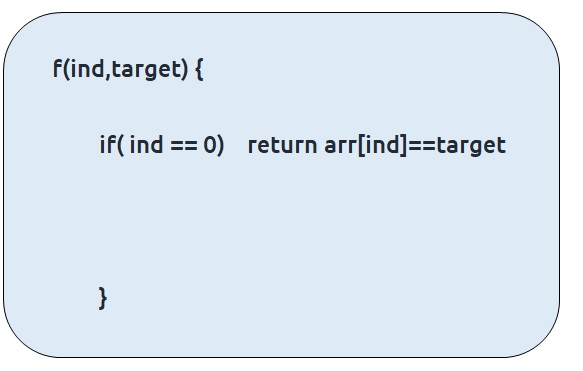• Now, the following cases can arise when we are at index 0, if the target sum is 0 and the first index is also 0, like in case [0,1], we can form the subset in two ways, either by considering the first element or leaving it, so we can return 2.• Else at index 0, if target == 0, and the first element is not 0, it means we will not pick the first element so we just return 1 way.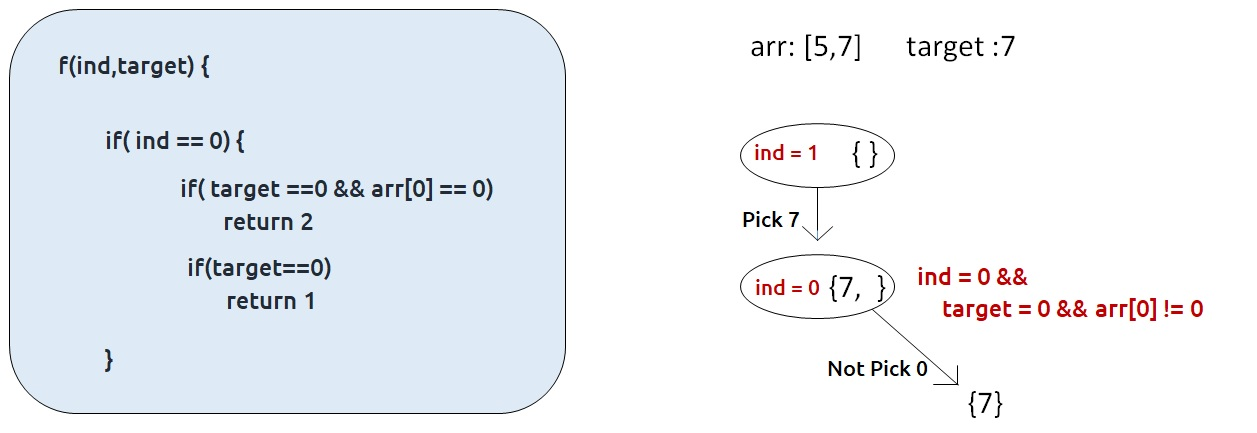• Or if at index 0, when the first element is not 0, and the target is equal to the first element , then we will include it in the subset and we will return 1 way.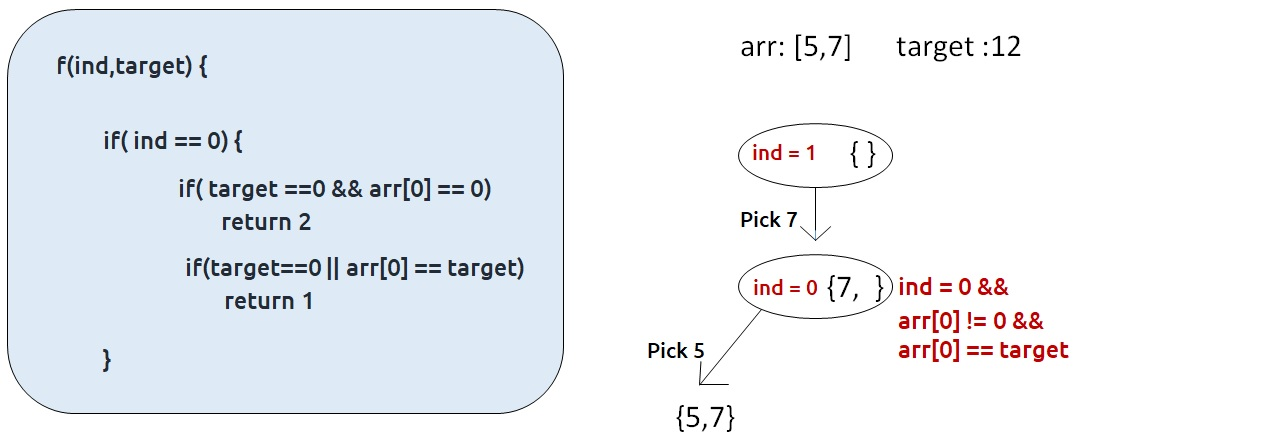• Else in all other cases, we simply return 0.

Code:

## C++ Code

``````#include <bits/stdc++.h>

using namespace std;

int findWaysUtil(int ind, int target, vector<int>& arr, vector<vector<int>> &dp){

if(ind == 0){
if(target==0 && arr==0)
return 2;
if(target==0 || target == arr)
return 1;
return 0;
}

if(dp[ind][target]!=-1)
return dp[ind][target];

int notTaken = findWaysUtil(ind-1,target,arr,dp);

int taken = 0;
if(arr[ind]<=target)
taken = findWaysUtil(ind-1,target-arr[ind],arr,dp);

return dp[ind][target]= notTaken + taken;
}

int findWays(vector<int> &num, int k){
int n = num.size();
vector<vector<int>> dp(n,vector<int>(k+1,-1));
return findWaysUtil(n-1,k,num,dp);
}

int main() {

vector<int> arr = {0,0,1};
int k=1;

cout<<"The number of subsets found are " <<findWays(arr,k);
}
``````

Output:

The number of subsets found are 4

## Java Code

``````import java.util.*;

class TUF{
static int findWaysUtil(int ind, int target,int[] arr, int[][] dp){

if(ind == 0){
if(target==0 && arr==0)
return 2;
if(target==0 || target == arr)
return 1;
return 0;
}

if(dp[ind][target]!=-1)
return dp[ind][target];

int notTaken = findWaysUtil(ind-1,target,arr,dp);

int taken = 0;
if(arr[ind]<=target)
taken = findWaysUtil(ind-1,target-arr[ind],arr,dp);

return dp[ind][target]= notTaken + taken;
}

static int findWays(int[] num, int k){
int n = num.length;
int dp[][]= new int[n][k+1];

for(int row[]: dp)
Arrays.fill(row,-1);

return findWaysUtil(n-1,k,num,dp);
}

public static void main(String args[]) {

int arr[] = {0,0,1};
int k=1;

System.out.println("The number of subsets found are "+findWays(arr,k));
}
}``````

Output:

The number of subsets found are 4

Part 2: Count Partitions with Given Difference

Problem Link: Partitions with Given Difference

Problem Description:

We are given an array ‘ARR’ with N positive integers and an integer D. We need to count the number of ways we can partition the given array into two subsets, S1 and S2 such that S1 – S2 = D and S1 is always greater than or equal to S2.

```Example: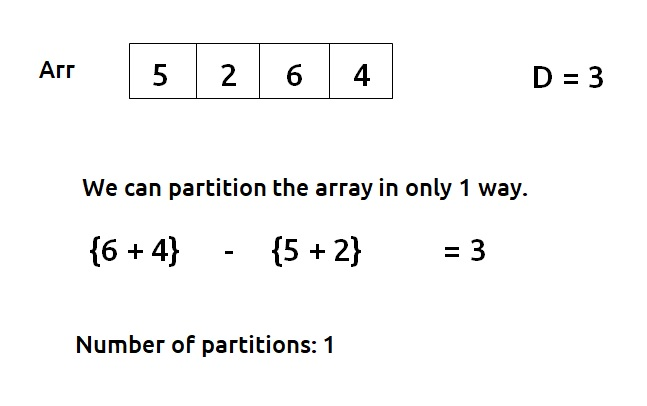```

Disclaimer: Don’t jump directly to the solution, try it out yourself first.

Pre-req: Count Subsets with Sum K

### Solution :

This question is a slight modification of the problem discussed in Count Subsets with Sum K.

We have the following two conditions given to us.

S1 + S2 = D   – (i)

S1 >= S2     – (ii)

If we calculate the total sum of elements of the array (say totSum), we can say that,

S1 = totSum – S2      – (iii)

Now solving for equations (i) and (iii), we can say that

S2 = (totSum – D)/2    – (iv)

Therefore the question “Count Partitions with a difference D” is modified to “Count Number of subsets with sum (totSum – D)/2 ”. This is exactly what we had discussed in the article  Count Subsets with Sum K.

Edge Cases:

The following edge cases need to be handled:

• As the array elements are positive integers including zero, we don’t want to find the case when S2 is negative or we can say that totSum is lesser than D, therefore if totSum<D, we simply return 0.
• S2 can’t be a fraction, as all elements are integers, therefore if totSum – D is odd, we can return 0.

From here on we will discuss the approach to “Count Subsets with Sum K” with the required modifications. Moreover, as the array elements can also contain 0, we will handle it as discussed in part-1 of this article.

Steps to form the recursive solution:

We will first form the recursive solution by the three points mentioned in Dynamic Programming Introduction

Step 1: Express the problem in terms of indexes.

The array will have an index but there is one more parameter “target”. We are given the initial problem to find whether there exists in the whole array a subsequence whose sum is equal to the target.

So, we can say that initially, we need to find(n-1, target) which means that we are counting the number of subsequences in the array from index 0 to n-1, whose sum is equal to the target. Similarly, we can generalize it for any index ind as follows: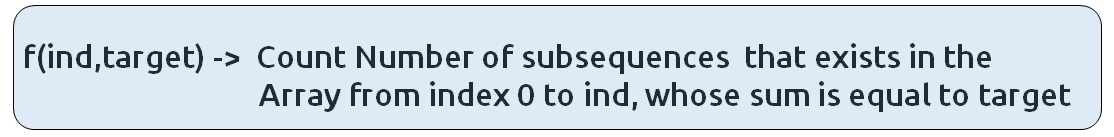Base Cases:

• If target == 0, it means that we have already found the subsequence from the previous steps, so we can return 1.
• If ind==0, it means we are at the first element, so we need to return arr[ind]==target. If the element is equal to the target we return 1 else we return 0.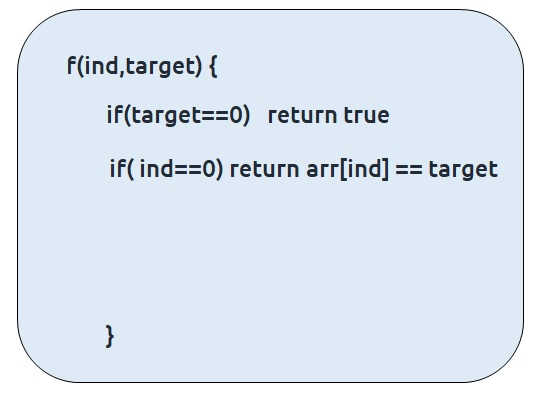Step 2: Try out all possible choices at a given index.

We need to generate all the subsequences. We will use the pick/non-pick technique as discussed in this video “Recursion on Subsequences”.

We have two choices:

• Exclude the current element in the subsequence: We first try to find a subsequence without considering the current index element. For this, we will make a recursive call to f(ind-1,target).
• Include the current element in the subsequence: We will try to find a subsequence by considering the current index as element as part of subsequence. As we have included arr[ind], the updated target which we need to find in the rest if the array will be target – arr[ind]. Therefore, we will call f(ind-1,target-arr[ind]).

Note: We will consider the current element in the subsequence only when the current element is less than or equal to the target.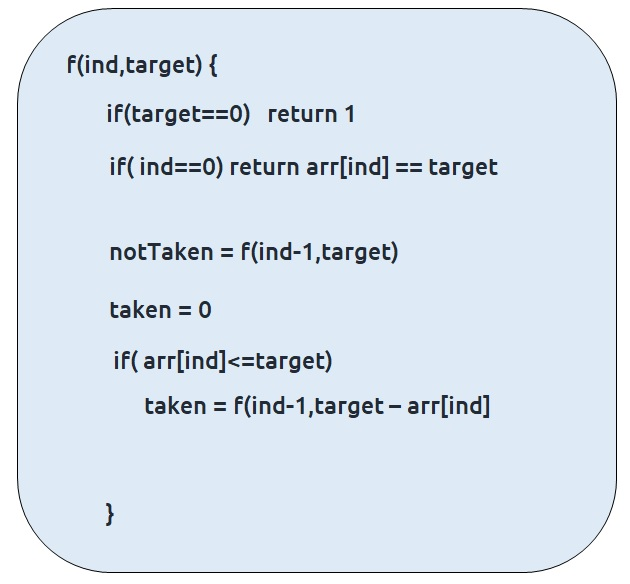Step 3:  Return sum of taken and notTaken

As we have to return the total count of subsets with the target sum, we will return the sum of taken and notTaken from our recursive call.

The final pseudocode after steps 1, 2, and 3:Steps to memoize a recursive solution:

If we draw the recursion tree, we will see that there are overlapping subproblems. In order to convert a recursive solution the following steps will be taken:

1. Create a dp array of size [n][k+1]. The size of the input array is ‘n’, so the index will always lie between ‘0’ and ‘n-1’. The target can take any value between ‘0’ and ‘k’. Therefore we take the dp array as dp[n][k+1]
2. We initialize the dp array to -1.
3. Whenever we want to find the answer of particular parameters (say f(ind,target)), we first check whether the answer is already calculated using the dp array(i.e dp[ind][target]!= -1 ). If yes, simply return the value from the dp array.
4. If not, then we are finding the answer for the given value for the first time, we will use the recursive relation as usual but before returning from the function, we will set dp[ind][target] to the solution we get.

Code:

## C++ Code

``````#include <bits/stdc++.h>

using namespace std;

int mod =(int)1e9+7;

int countPartitionsUtil(int ind, int target, vector<int>& arr, vector<vector
<int>> &dp){

if(ind == 0){
if(target==0 && arr==0)
return 2;
if(target==0 || target == arr)
return 1;
return 0;
}

if(dp[ind][target]!=-1)
return dp[ind][target];

int notTaken = countPartitionsUtil(ind-1,target,arr,dp);

int taken = 0;
if(arr[ind]<=target)
taken = countPartitionsUtil(ind-1,target-arr[ind],arr,dp);

return dp[ind][target]= (notTaken + taken)%mod;
}

int countPartitions(int d, vector<int>& arr){
int n = arr.size();
int totSum = 0;
for(int i=0; i<arr.size();i++){
totSum += arr[i];
}

//Checking for edge cases
if(totSum-d<0) return 0;
if((totSum-d)%2==1) return 0;

int s2 = (totSum-d)/2;

vector<vector<int>> dp(n,vector<int>(s2+1,-1));
return countPartitionsUtil(n-1,s2,arr,dp);
}

int main() {

vector<int> arr = {5,2,6,4};
int d=3;

cout<<"The number of subsets found are " <<countPartitions(d,arr);
}
``````

Output:

The number of subsets found are 1

Time Complexity: O(N*K)

Reason: There are N*K states therefore at max ‘N*K’ new problems will be solved.

Space Complexity: O(N*K) + O(N)

Reason: We are using a recursion stack space(O(N)) and a 2D array ( O(N*K)).

## Java Code

``````import java.util.*;

class TUF{

static int mod =(int)(Math.pow(10,9)+7);
static int countPartitionsUtil(int ind, int target,int[] arr, int[][] dp){

if(ind == 0){
if(target==0 && arr==0)
return 2;
if(target==0 || target == arr)
return 1;
return 0;
}

if(dp[ind][target]!=-1)
return dp[ind][target];

int notTaken = countPartitionsUtil(ind-1,target,arr,dp);

int taken = 0;
if(arr[ind]<=target)
taken = countPartitionsUtil(ind-1,target-arr[ind],arr,dp);

return dp[ind][target]= (notTaken + taken)%mod;
}

static int countPartitions(int d,int[] arr){
int n = arr.length;
int totSum = 0;
for(int i=0; i<arr.length;i++){
totSum += arr[i];
}

//Checking for edge cases
if(totSum-d<0) return 0;
if((totSum-d)%2==1) return 0;

int s2 = (totSum-d)/2;

int dp[][] = new int[n][s2+1];

for(int row[]: dp)
Arrays.fill(row,-1);

return countPartitionsUtil(n-1,s2,arr,dp);
}

public static void main(String args[]) {

int arr[] = {5,2,6,4};
int d=3;

System.out.println("The number of subsets found are "+countPartitions(d,arr));
}
}``````

Output:

The number of subsets found are 1

Time Complexity: O(N*K)

Reason: There are N*K states therefore at max ‘N*K’ new problems will be solved.

Space Complexity: O(N*K) + O(N)

Reason: We are using a recursion stack space(O(N)) and a 2D array ( O(N*K)).

Steps to convert Recursive Solution to Tabulation one.

To convert the memoization approach to a tabulation one, create a dp array with the same size as done in memoization. We can initialize it as 0.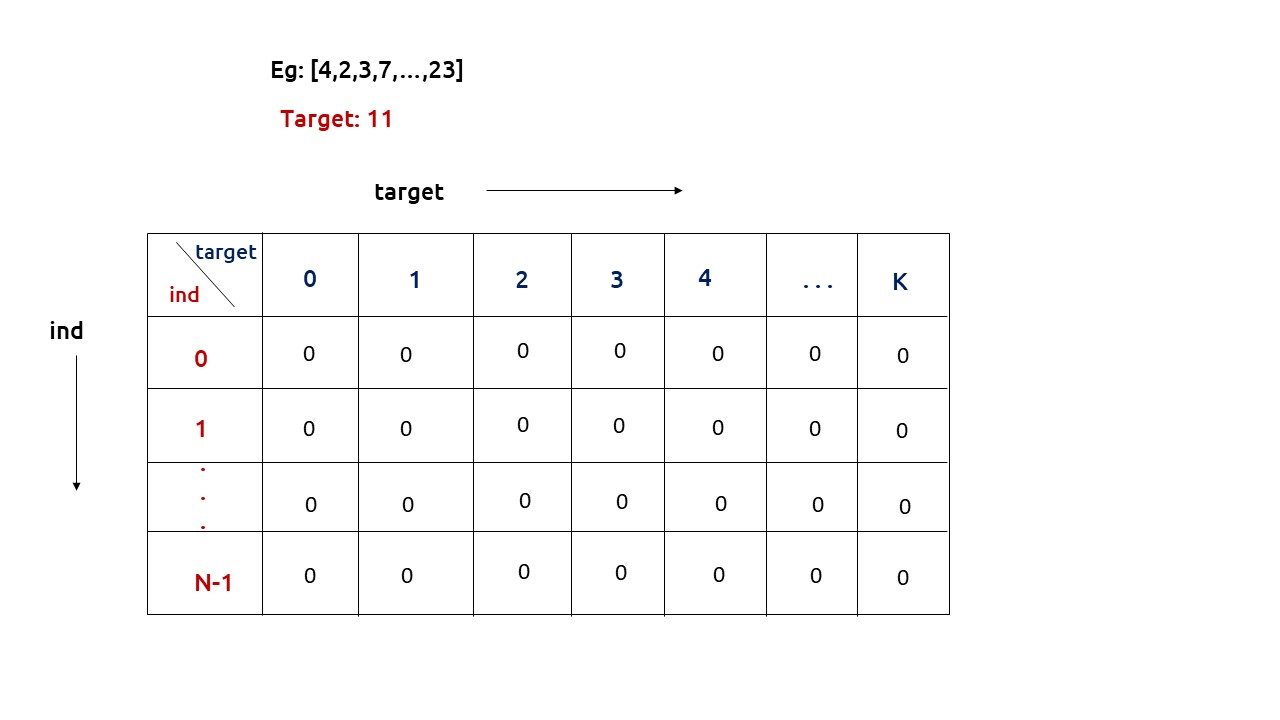First, we need to initialize the base conditions of the recursive solution.

• If target == 0, ind can take any value from 0 to n-1, therefore we need to set the value of the first column as 1.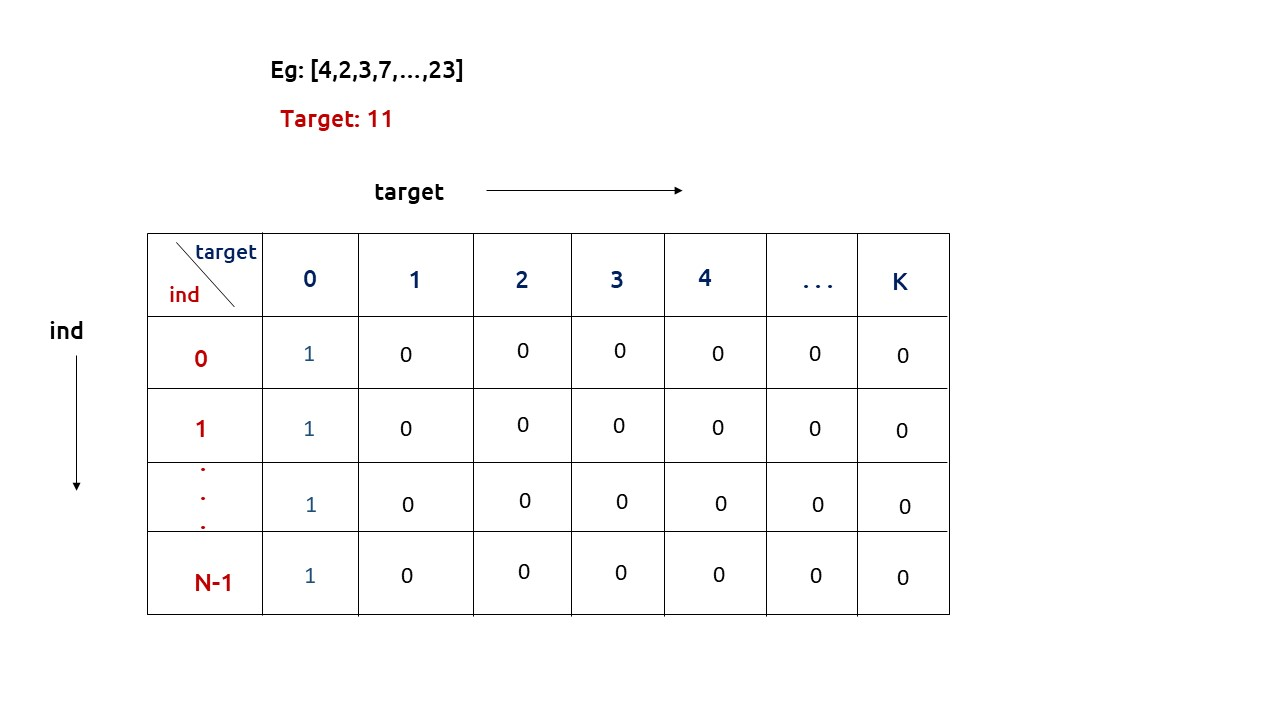• The first row dp[] indicates that only the first element of the array is considered, therefore for the target value equal to arr, only cell with that target will be true, so explicitly set dp[arr] =1, (dp[arr] means that we are considering the first element of the array with the target equal to the first element itself). Please note that it can happen that arr>target, so we first check it: if(arr<=target) then set dp[arr] = 1.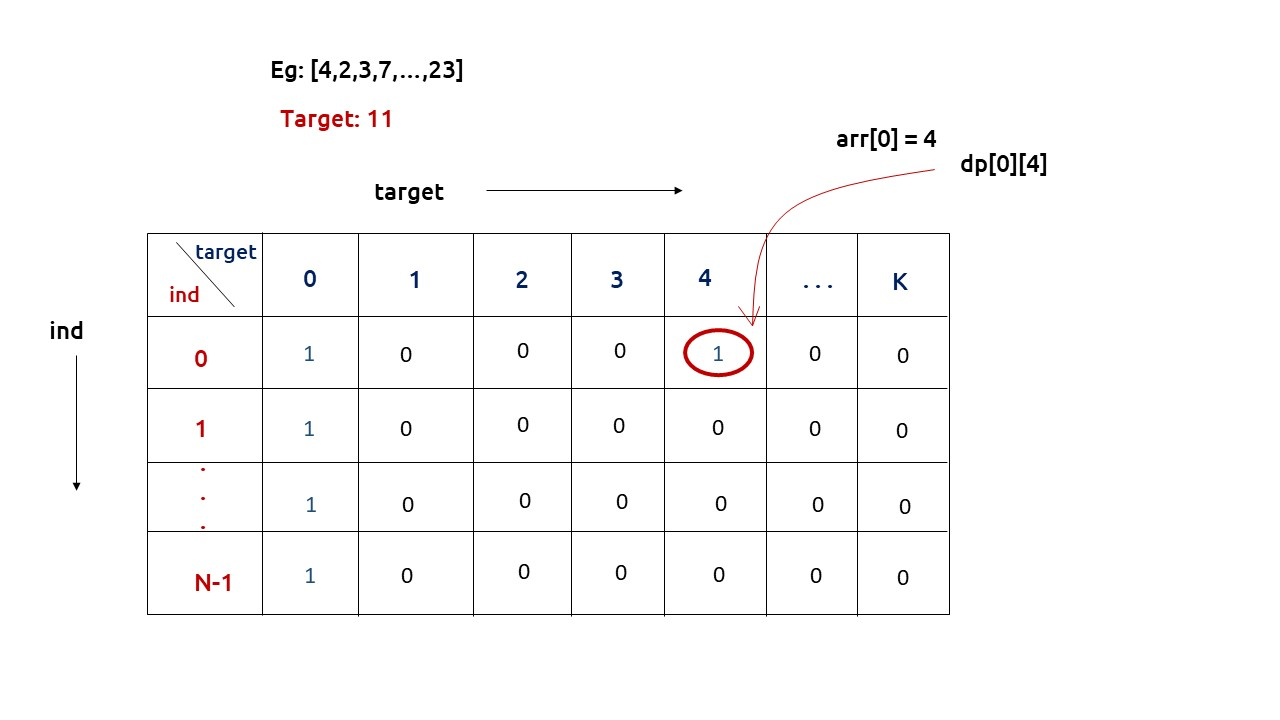• After that , we will set our nested for loops to traverse the dp array and following the logic discussed in the recursive approach, we will set the value of each cell. Instead of recursive calls, we will use the dp array itself.
• At last we will return dp[n-1][k] as our answer.

Code:

## C++ Code

``````#include <bits/stdc++.h>

using namespace std;

int mod =(int)1e9+7;

int findWays(vector<int> &num, int tar){
int n = num.size();

vector<vector<int>> dp(n,vector<int>(tar+1,0));

if(num == 0) dp =2;  // 2 cases -pick and not pick
else dp = 1;  // 1 case - not pick

if(num!=0 && num<=tar) dp[num] = 1;  // 1 case -pick

for(int ind = 1; ind<n; ind++){
for(int target= 0; target<=tar; target++){

int notTaken = dp[ind-1][target];

int taken = 0;
if(num[ind]<=target)
taken = dp[ind-1][target-num[ind]];

dp[ind][target]= (notTaken + taken)%mod;
}
}
return dp[n-1][tar];
}

int countPartitions(int n, int d, vector<int>& arr){
int totSum = 0;
for(int i=0; i<n;i++){
totSum += arr[i];
}

//Checking for edge cases
if(totSum-d <0 || (totSum-d)%2 ) return 0;

return findWays(arr,(totSum-d)/2);
}

int main() {

vector<int> arr = {5,2,6,4};
int n = arr.size();
int d=3;

cout<<"The number of subsets found are " <<countPartitions(n,d,arr);
}
``````

Output:

The number of subsets found are 1

Time Complexity: O(N*K)

Reason: There are two nested loops

Space Complexity: O(N*K)

Reason: We are using an external array of size ‘N*K’. Stack Space is eliminated.

## Java Code

``````import java.util.*;

class TUF{
static int mod =(int)(Math.pow(10,9)+7);

static int findWays(int[] num, int tar){
int n = num.length;

int dp[][] = new int[n][tar+1];

if(num == 0) dp =2;  // 2 cases -pick and not pick
else dp = 1;  // 1 case - not pick

if(num!=0 && num<=tar) dp[num] = 1;  // 1 case -pick

for(int ind = 1; ind<n; ind++){
for(int target= 0; target<=tar; target++){

int notTaken = dp[ind-1][target];

int taken = 0;
if(num[ind]<=target)
taken = dp[ind-1][target-num[ind]];

dp[ind][target]= (notTaken + taken)%mod;
}
}
return dp[n-1][tar];
}

static int countPartitions(int n, int d,int[] arr){
int totSum = 0;
for(int i=0; i<n;i++){
totSum += arr[i];
}

//Checking for edge cases
if(totSum-d <0 || (totSum-d)%2==1 ) return 0;

return findWays(arr,(totSum-d)/2);
}

public static void main(String args[]) {

int arr[] = {5,2,6,4};
int n = arr.length;
int d=3;

System.out.println("The number of subsets found are "+countPartitions(n,d,arr));
}
}``````

Output:

The number of subsets found are 1

Time Complexity: O(N*K)

Reason: There are two nested loops

Space Complexity: O(N*K)

Reason: We are using an external array of size ‘N*K’. Stack Space is eliminated.

Part 3: Space Optimization

If we closely look the relation,

dp[ind][target] =  dp[ind-1][target] + dp[ind-1][target-arr[ind]]

We see that to calculate a value of a cell of the dp array, we need only the previous row values (say prev). So, we don’t need to store an entire array. Hence we can space optimize it.

Note: Whenever we create a new row ( say cur), we need to explicitly set its first element is true according to our base condition.

Code:

## C++ Code

``````#include <bits/stdc++.h>

using namespace std;

int mod =(int)1e9+7;

int findWays(vector<int> &num, int tar){
int n = num.size();

vector<int> prev(tar+1,0);

if(num == 0) prev =2;  // 2 cases -pick and not pick
else prev = 1;  // 1 case - not pick

if(num!=0 && num<=tar) prev[num] = 1;  // 1 case -pick

for(int ind = 1; ind<n; ind++){
vector<int> cur(tar+1,0);
for(int target= 0; target<=tar; target++){
int notTaken = prev[target];

int taken = 0;
if(num[ind]<=target)
taken = prev[target-num[ind]];

cur[target]= (notTaken + taken)%mod;
}
prev = cur;
}
return prev[tar];
}

int countPartitions(int n, int d, vector<int>& arr){
int totSum = 0;
for(int i=0; i<n;i++){
totSum += arr[i];
}

//Checking for edge cases
if(totSum-d <0 || (totSum-d)%2 ) return 0;

return findWays(arr,(totSum-d)/2);
}

int main() {

vector<int> arr = {5,2,6,4};
int n = arr.size();
int d=3;

cout<<"The number of subsets found are " <<countPartitions(n,d,arr);
}
``````

Output:

The number of subsets found are 1

Time Complexity: O(N*K)

Reason: There are three nested loops

Space Complexity: O(K)

Reason: We are using an external array of size ‘K+1’ to store only one row.

## Java Code

``````import java.util.*;

class TUF{

static int mod =(int)(Math.pow(10,9)+7);

static int findWays(int[] num, int tar){
int n = num.length;

int prev[] = new int[tar+1];

if(num == 0) prev =2;  // 2 cases -pick and not pick
else prev = 1;  // 1 case - not pick

if(num!=0 && num<=tar) prev[num] = 1;  // 1 case -pick

for(int ind = 1; ind<n; ind++){
int cur[]=new int[tar+1];
for(int target= 0; target<=tar; target++){
int notTaken = prev[target];

int taken = 0;
if(num[ind]<=target)
taken = prev[target-num[ind]];

cur[target]= (notTaken + taken)%mod;
}
prev = cur;
}
return prev[tar];
}

static int countPartitions(int n, int d,int[] arr){
int totSum = 0;
for(int i=0; i<n;i++){
totSum += arr[i];
}

//Checking for edge cases
if(totSum-d <0 || (totSum-d)%2 ==1) return 0;

return findWays(arr,(totSum-d)/2);
}

public static void main(String args[]) {

int arr[] = {5,2,6,4};
int n = arr.length;
int d=3;

System.out.println("The number of subsets found are " +countPartitions(n,d,arr));
}
}``````

Output:

The number of subsets found are 1

Time Complexity: O(N*K)

Reason: There are three nested loops

Space Complexity: O(K)

Reason: We are using an external array of size ‘K+1’ to store only one row.

Special thanks to Anshuman Sharma for contributing to this article on takeUforward. If you also wish to share your knowledge with the takeUforward fam, please check out this article